Learn you an Agda
DEPRECATED: Propositions and Predicates
16th Febuary 2011

Warning: This page is still under development. Check back later for a completed version

# “Logic is the art of going wrong with confidence”

Now that we’ve defined the natural numbers, we’re going to do some simple example proofs of some basic mathematical properties. We’ll first discuss logic and logic specification in natural deduction, and as we go, we’ll discuss the application to Agda.

At a fundamental level, a logic is a system of judgements. Judgements are statements in a mathematical language that may be proven, or unproven. A language is usually described as a set of strings, which make up every term in the language, but this is a simplification: a language can be made up of arbitrary data structures. We just use strings to represent these structures because any data structure can be represented in some string form. We’re going to look at some basic logics, and then encode them within Agda, using the Curry-Howard correspondence.

For our example, we will define a very simple system based on the language of natural numbers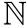we used earlier.

We’re going to have just one type of judgement, of the form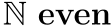, which is provable only when the given number is even.

A logic consists of a set of axioms, and a set of rules. Axioms are the foundation of the logic: they’re the basic, simple statements that are assumed to be true. Rules describe how to produce new proven statements, or theorems, given some existing theorems. We can formally specify these axioms and rules in a meta-logic called natural deduction, by writing them in the form of inference rules, which look like this: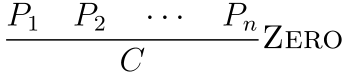This says that if we can prove all of the premises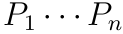, then we can prove the conclusion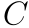.

For our purposes, we have just one axiom, that the number zero is even. Axioms are written as inference rules with no premises: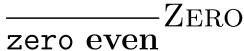Then, based on the inductive reasoning we used earlier, our rules for our system need to express that if some number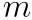is even, then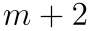is also even. We do this by writing an inference rule schema, which is a way of describing a set of rules, by including one or more metavariables in an inference rule. If I have some metavariable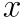in a rule schema, I can substitutefor any wellformed term in the language, and I will have a valid rule.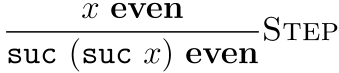If I wanted to show that four was even, I would be able to apply this rule twice (once whereis two, and once whenis zero), leaving the obligation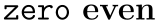which is shown by the axiom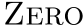. We can write this proof using natural deduction in a “proof tree” format: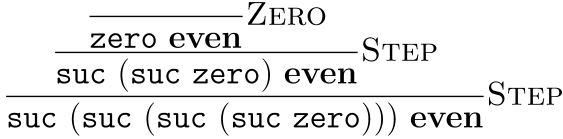When proving a theorem, we work from the bottom of this tree upwards, applying rules that fit the form of the goal as we go. When reading the proof, we work downwards, reasoning from known axioms to the theorem that we want.

# How does this all relate to Agda?

As we’re embedding these logics inside Agda, Agda’s types correspond to their judgements. Remember that if we can construct a value of a certain type, we have simultaneously constructed a proof that the theorem encoded by that type holds. As types are judgements in our embedding, and values are their proofs, data constructors for a type correspond to inference rules in the system. Let’s encode the judgement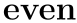in Agda, based on our definition in natural deduction.

We’ll use the mix-fix name _even here rather than just even so that we can use the judgement in post-fix form. As our judgement is over the language of natural numbers, we index the type constructor for our judgement by the type ℕ.

data _even : ℕ → Set where

This allows us to define the axiomas a simple constructor for the type zero even:

   ZERO : zero even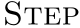is a little more complicated however, due to the presence of the metavariable. Just writing the rule as-is will result in an error, asis not in scope:

   STEP : x even → suc (suc x) even -- x not in scope

To solve this, we make STEP take a dependent parameter, a natural number:

   STEP : (x : ℕ) → x even → suc (suc x) even

By using dependent types, we can define rule schema: STEP zero here refers to the rule zero even → suc (suc zero) even, which shows that STEP the same substitution semantics that we described for metavariables earlier.

In this case, the type of x can be inferred from its usage, as we use it with _even which takes an ℕ as an argument, so we can use the special ∀ symbol to introduce x and omit the type:

   STEP : ∀ x → x even → suc (suc x) even

So, our final definition of _even is:

data _even : ℕ → Set where
ZERO : zero even
STEP : ∀ x → x even → suc (suc x) even

Now we’ll prove in Agda that four is even. Type the following into an emacs buffer, and type C-c C-l:

-- \_1 to type ₁
proof₁ : suc (suc (suc (suc zero))) even
proof₁ = ?

Agda will show a small “hole” in the code, which looks like this:

proof₁ : suc (suc (suc (suc zero))) even
proof₁ = { }0

and in a separate window will be shown:

?0 : suc (suc (suc (suc zero))) even

This tells us that our proof obligation at hole ?0 is suc (suc (suc (suc zero))) even. Next, put your cursor into the hole, and type STEP ? ? into it, then type C-c C-space. This will split your hole into two more holes: ?1 is the number to provide for x in STEP, and ?2 is a proof obligation to show that two is even:

proof₁ : suc (suc (suc (suc zero))) even
proof₁ = STEP { }1 { }2

In this case, there is only one constructor for hole ?2 that fits — STEP, so we can just type C-c C-r in that hole and Agda will split it into another STEP call for us, resulting in two more holes ?3 and ?4:

proof₁ : suc (suc (suc (suc zero))) even
proof₁ = STEP { }1 (STEP { }3 { }4)

C-c C-r will happilly fill in ZERO for us in hole ?4, and the remaining two holes, ?1 and ?3, are already known to Agda from the surrounding context. We can see what constraints Agda knows about on our holes by using the “Show Constraints” option or typing C-c C-=. This will print out:

?1 := suc (suc zero)
?3 := zero

Seeing as this is the case, we can have Agda fill in the holes for us by using the “Solve Constraints” option or C-c C-s. Our final proof becomes:

proof₁ : suc (suc (suc (suc zero))) even
proof₁ = STEP (suc (suc zero)) (STEP zero ZERO)

Another feature worth mentioning is Agsy, an automatic proof searcher for Agda. Simply type C-c C-a in any hole and Agsy will search for an appropriate term to fill it. It’s not guaranteed to find anything, but it can be useful (it works well in this case).

## Implicits

It can be annoying, though, to have to pass in those numbers to STEP explicitly, when Agda already knows from surrounding context exactly what they are. In these situations, you can use a single underscore (_) to indicate that you wish Agda to infer the value in this position during typechecking.

proof₁ : suc (suc (suc (suc zero))) even
proof₁ = STEP _ (STEP _ ZERO)

If Agda cannot infer the value of the implicit underscore, an “unsolved metavariable” will be shown in the goals window, and the underscore will be highlighted in yellow.

For this particular judgement, however, it is almost always obvious from known constraints what the value of those numbers should be. In these cases, it’s common to use an implicit parameter when declaring the type:

data _even : ℕ → Set where
ZERO : zero even
STEP : ∀ {x} → x even → suc (suc x) even    -- long form { x : ℕ } is also fine

Note that here we have added braces around the variable x in our definition of STEP. This lets us omit the number entirely when writing our proof:

proof₁ : suc (suc (suc (suc zero))) even
proof₁ = STEP (STEP ZERO)

If Agda cannot, for whatever reason, infer the value of the implicit parameter, yellow highlighting and an unsolved metavariable will be added, as before. In those scenarios, you can manually specify the value of the implicit parameter by using braces:

proof₁ : suc (suc (suc (suc zero))) even
proof₁ = STEP {suc (suc zero)} (STEP {zero} ZERO)

For _even, this is not usually necessary, but if you find yourself specifying implicit parameters frequently, you may wish to consider making it explicit.

TODO: REVISE

## Implication

What if we wanted to talk about more interesting propositions in Agda? If we wanted to show an implication, for example? In Agda, an implication proposition corresponds to a function type.

When we prove an implication, say A ⇒ B, we assume the premise (A) to be true, and from there try and derive the conclusion (B). If we think in terms of proof by construction, this is the same as implementing a function - a function, when given an argument of type A, constructs an return value of type B. In other words, when given a proof (by construction) of some proposition A, our function will produce a proof of some proposition B - This is exactly the same as our proof method for implication! If we can write such a function (and Agda checks it), then simply by writing that function we have constructed a proof of A ⇒ B.

Consider a simple statement that is tautologically true: A ⇒ A (or, if A is true, then A is true). Let’s prove this obvious statement in Agda!

First, let’s prove it just for the proposition that 2 is even:


proof₂ : (suc (suc zero)) even → (suc (suc zero)) even
proof₂ ν = ν      -- \nu to type ν.


So, this tautology proof corresponds to the identity function. The reason for this is fairly clear: Given a proof of A, there’s only one real way to produce a proof of A, and that is to produce the proof you were just given.

## Universal Quantification

It would be nice though, to make this proof about all propositions, not merely the proposition that natural numbers exist - after all, the proof is the same regardless of the proposition involved!

To do this, we have to exploit Agda’s flexible type system a little. We make our identity function take an additional parameter - a type. Given a type, we then return an identity function, instantiated for that type.

proof₂′ : (A : Set) → A → A
proof₂′ _ x = x

The new type signature here means: Given some value of type Set (i.e a type), called A, this returns a function from A to A. Alternatively, we could view it as a logical statement: For any proposition A, A ⇒ A. In logic, we use the symbol ∀ to mean “for any” or “for all”. So, the above type signature could be written in logic as:

$$\forall a. A \Rightarrow A$$

Making propositions about all members of a set (or universe) is called Universal Quantification, and it corresponds to parametric polymorphism (including Java generics and C++ templates) in type system lingo.

Now we can implement our special case proof in terms of the more general one:

proof₂ : (suc (suc zero)) even → (suc (suc zero)) even
proof₂ = proof₂′ ℕ

## Conjunction

Unlike universal quantification or implication, Conjunction and disjunction do not correspond to built-in types in Agda, however they are fairly straightforward to define.

When we prove a conjunction on pen and paper, we simply prove both of the two components of the conjunction. If we have a proof for both components, then we automatically have a proof of a conjunction. This means that conjunction corresponds to a pair or a tuple (more formally known as a product type) in Agda.

data _∧_ (P : Set) (Q : Set) : Set where -- \and for ∧
∧-intro : P → Q → (P ∧ Q)

Here we’ve defined a new data type, this time it is parameterized by two types/propositions, which make up the components of the conjunction. Conjunction itself is also a proposition, so we give it the type Set.

Notice how the ∧-intro constructor can only produce a proof of P ∧ Q if it is passed both a proof of P and a proof of Q. This is how conjunction is demonstrated by construction - it is impossible to create a conjunction that is not supported by proofs for both components.

Using this we can prove some simple properties about conjunctions, such as: P ∧ Q ⇒ P:

proof₃ : {P Q : Set} → (P ∧ Q) → P
proof₃ (∧-intro p q) = p

## Equivalence

Now that we have defined conjunction and implication, we can define a notion of logical equivalence. Two propositions are equivalent if they imply each other. This is defined as: if one is true, the other is also true.

_⇔_ : (P : Set) → (Q : Set) → Set -- \<=> to type ⇔
a ⇔ b = (a → b) ∧ (b → a)

## Serious Proofs

Using this we can come up with some proofs of the algebraic properties of conjunction. The commutative property says that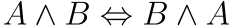, i.e the order of conjuncts does not matter. Let’s prove it:

∧-comm′ : {P Q : Set} → (P ∧ Q) → (Q ∧ P)
∧-comm′ (∧-intro p q) = ∧-intro q p

∧-comm : {P Q : Set} → (P ∧ Q) ⇔ (Q ∧ P)
∧-comm = ∧-intro (∧-comm′ {P} {Q}) (∧-comm′ {Q} {P}) -- implicits provided for clarity only.

Let’s also prove associativity (i.e that brackets don’t matter):

∧-assoc₁ : { P Q R : Set } → ((P ∧ Q) ∧ R) → (P ∧ (Q ∧ R))
∧-assoc₁ (∧-intro (∧-intro p q) r) = ∧-intro p (∧-intro q r)

∧-assoc₂ : { P Q R : Set } → (P ∧ (Q ∧ R)) → ((P ∧ Q) ∧ R)
∧-assoc₂ (∧-intro p (∧-intro q r)) = ∧-intro (∧-intro p q) r

∧-assoc : { P Q R : Set } → ((P ∧ Q) ∧ R) ⇔  (P ∧ (Q ∧ R))
∧-assoc = ∧-intro ∧-assoc₁ ∧-assoc₂

## Disjunction

If conjunction is a pair, because it requires both proofs to hold, then disjunction is a sum type (also known as an Either type), because it only requires one proof in order to hold. In order to model this in Agda, we add two constructors to the type, one for each possible component of the disjunction.

data _∨_ (P Q : Set) : Set where
∨-intro₁ : P → P ∨ Q
∨-intro₂ : Q → P ∨ Q

Using this, we can come up with some interesting proofs. The simplest one to prove is disjunction elimination, which is: ∀A B C ⇒ ((A ⇒ C) ∧ (B ⇒ C) ∧ (A ∨ B))⇒ C. In plain English, this means “If I know that C is true if A is true, and that C is true if B is true, and that A or B is true, then I know C is true”.

∨-elim : {A B C : Set} → (A → C) → (B → C) → (A ∨ B) → C
∨-elim ac bc (∨-intro₁ a) = ac a
∨-elim ac bc (∨-intro₂ b) = bc b

We can also prove the algebraic properties of disjunction, such as commutativity:

∨-comm′ : {P Q : Set} → (P ∨ Q) → (Q ∨ P)
∨-comm′ (∨-intro₁ p) = ∨-intro₂ p
∨-comm′ (∨-intro₂ q) = ∨-intro₁ q

∨-comm : {P Q : Set} → (P ∨ Q) ⇔ (Q ∨ P)
∨-comm = ∧-intro ∨-comm′ ∨-comm′

The associativity proof is left as an exercise to the reader1.

## Negation

The only false propositions that exist in logic are values for which there can exist no proof. Therefore, the only way to prove that a proposition is false is to show that the corresponding type contains no values.

In Agda, the basic type for false propositions is usually called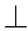, or “bottom”, written \bot.

data ⊥ : Set where -- nothing

That’s right. No, it’s not a mistake. There are no constructors for ⊥!

Having such a value allows us to define negation (¬A) as true if A being true would mean bottom is true (which is impossible). In other words, we can say that the type A has no inhabitants if every inhabitant of A can be mapped to an inhabitant of ⊥. As there are no inhabitants of ⊥, the only way this can be true is if A also has no inhabitants.

¬ : Set → Set -- for ¬ type \neg
¬ A = A → ⊥   

## Can’t get something from nothing

Agda’s logic is constructive, which means it’s impossible to prove the statement that all statements are either true or false,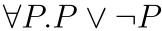. This proposition is called the law of the excluded middle. This makes it impossible to perform, for example, a general proof by contradiction, or other similar “classical” proofs from mathematics. There is a large philosophical debate among mathematicians (those who follow Hilbert, and those who follow Brouwer) as to which is better.

# The Curry Howard Correspondence

This chapter has taught you how to encode propositional logic into Agda’s type system. The correspondences discussed here between disjunction and sum types, conjunction and product types, functions and implication, and propositions and types are the fundamentals behind the Curry Howard correspondence. Using these tools, you can encode any constructive proof in Agda, which covers a vast range of possible proofs, including the vast majority of proofs encountered in program verification.

The next chapter will introduce relational equality, and begin proving some theorems about the Peano numbers we introduced in the previous chapter. Happy Hacking!

1. Muahahaha.